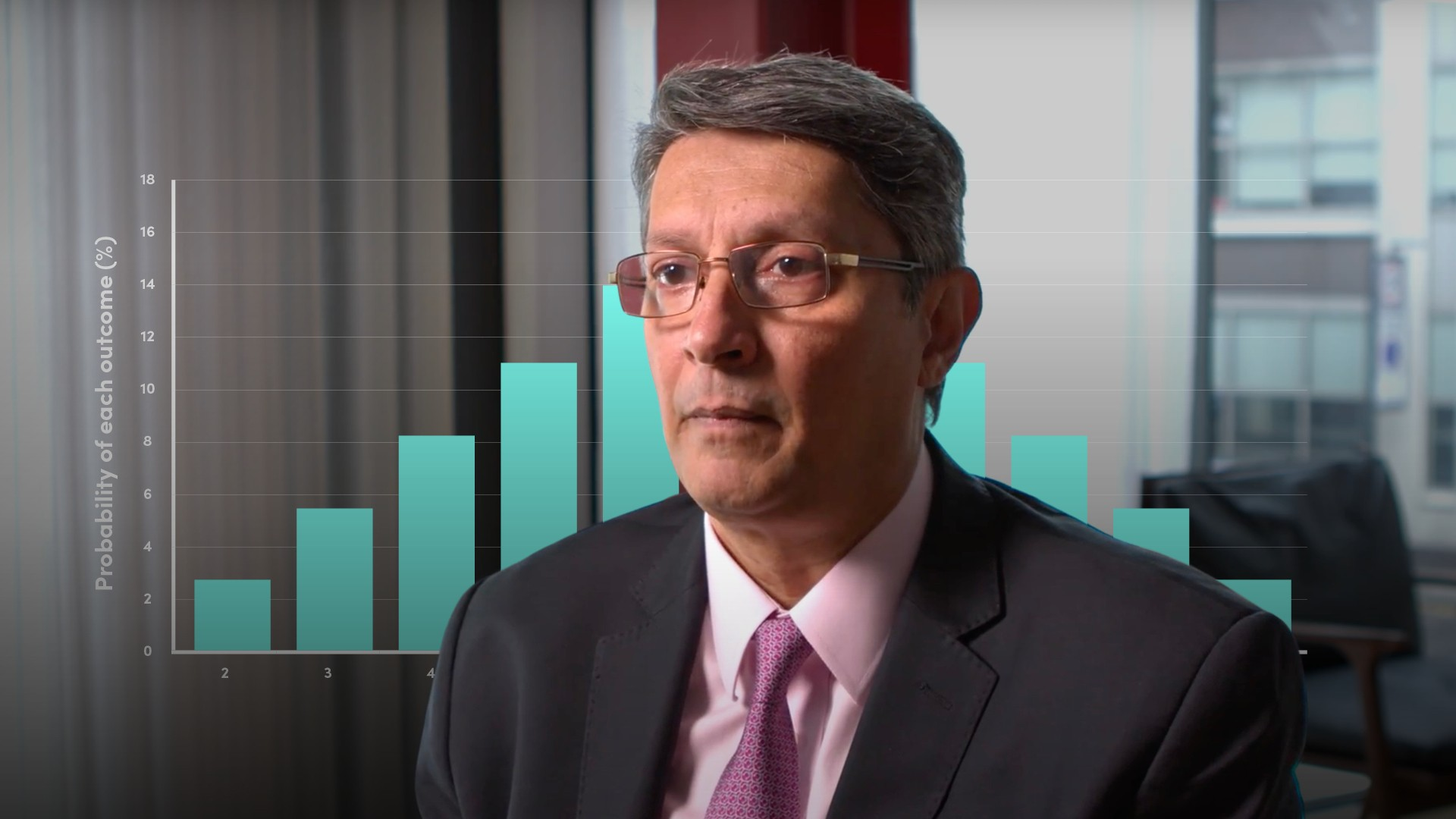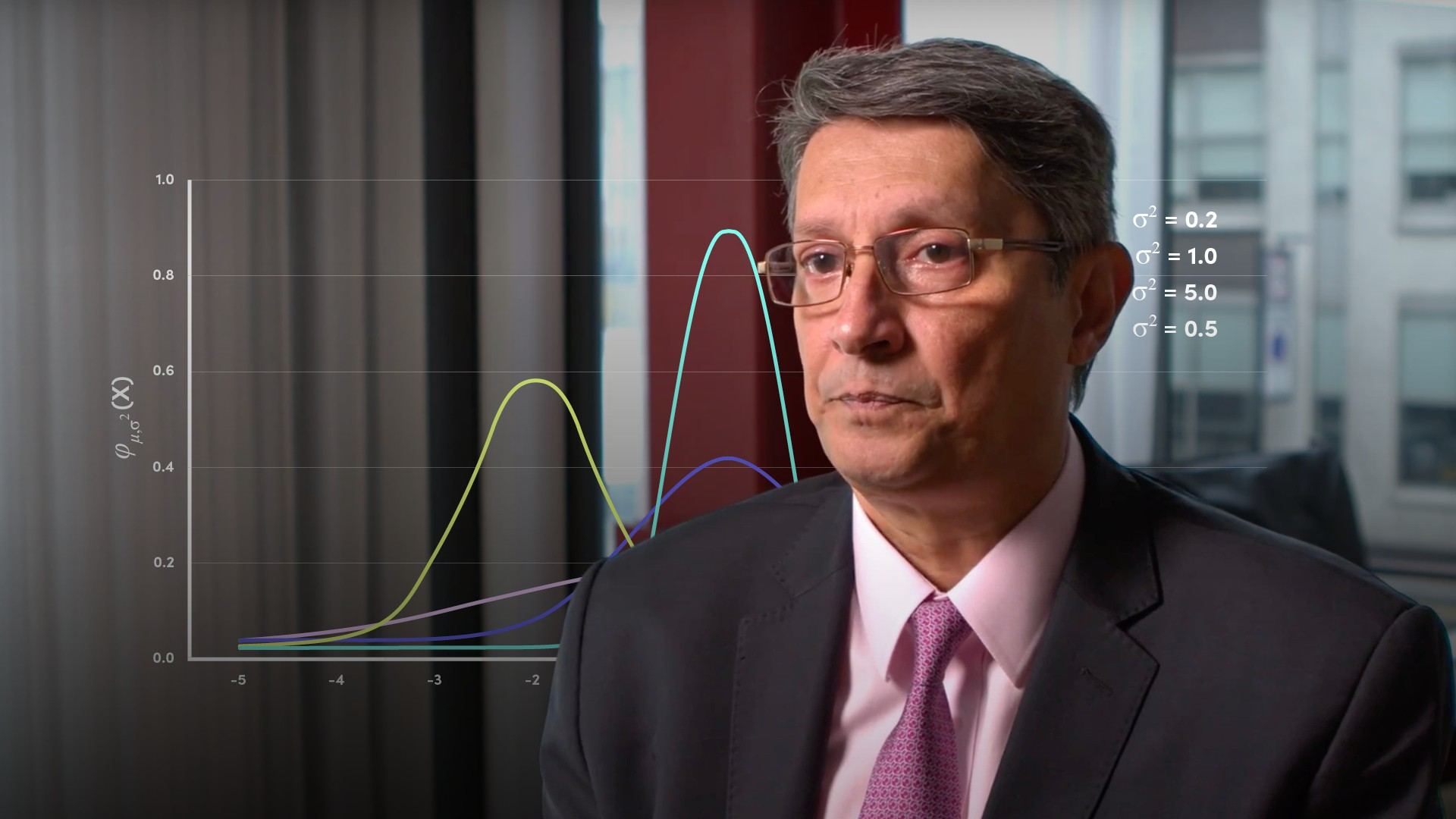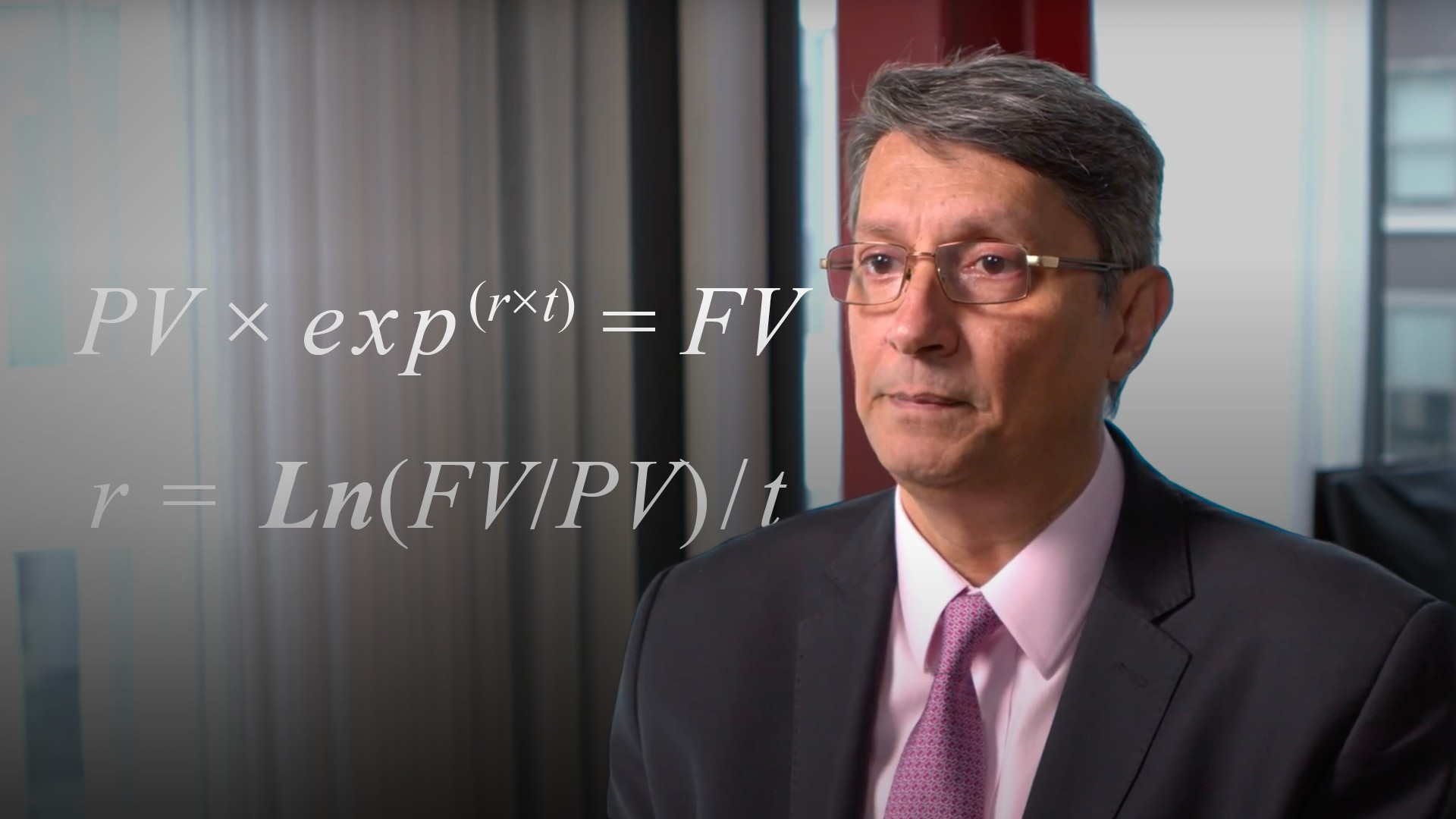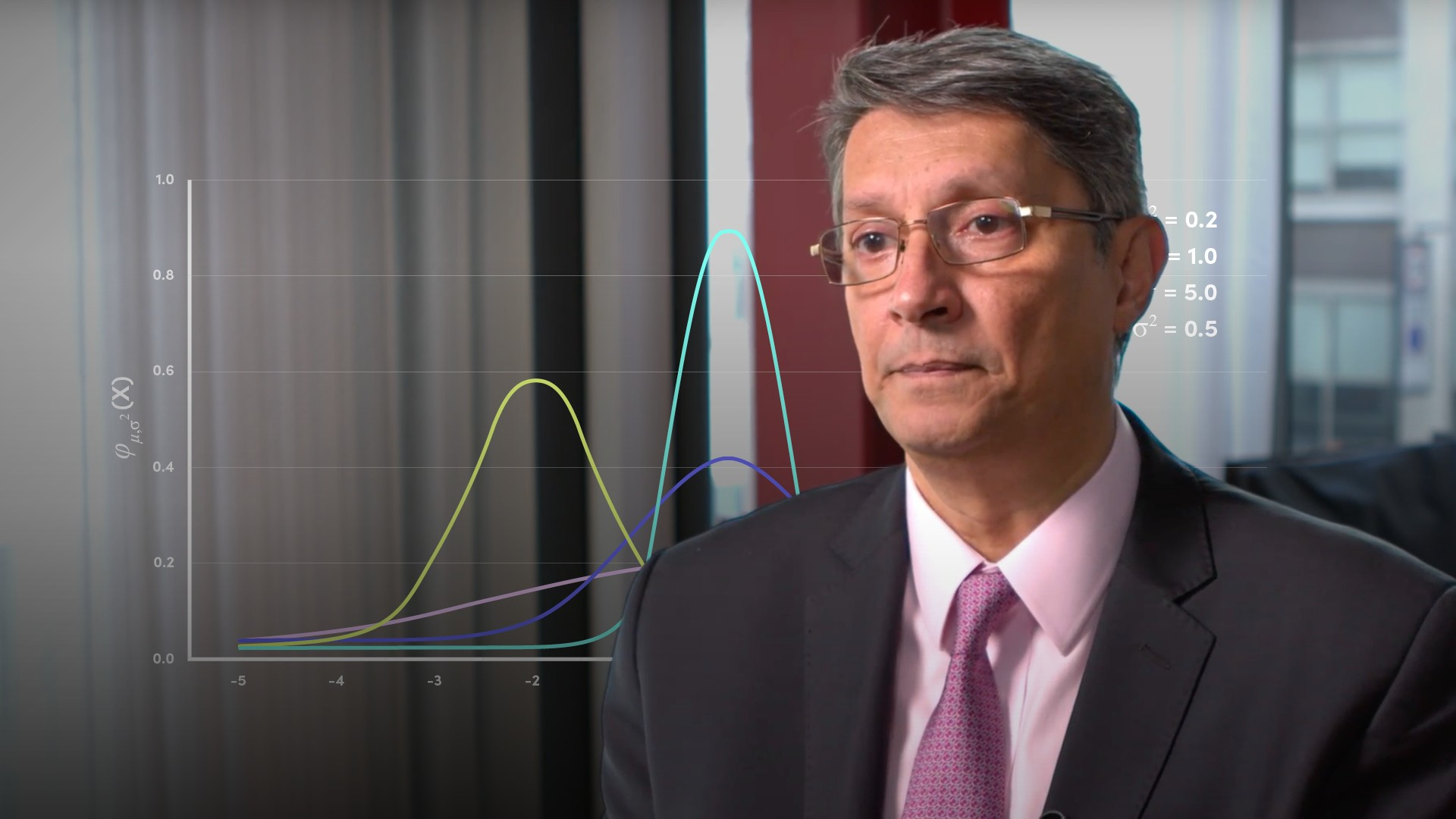# Probability Distributions: Financial Maths Part III

In their simplest form, probability distributions indicate the likelihood of an outcome or an event. In turn, investors use probability distributions to calculate returns on assets and potential catastrophic events so that they can understand and then hedge their risk. This pathway will explore the basics, and then some valuable forms of probability distributions that you should know about.

Pathway

Technical FoundationsAccess this and 100s of other videos by signing up for a 14-day free trial.

#### CPD credits earned

1#### Certificates

Watch all the videos and pass the test to obtain a certificate showing your completion of this Pathway. Certificates can be shared directly to your LinkedIn profile and social media accounts.

7 videos • 33 minutes

•Financial maths### The Basics of Probability Distributions

In financial markets the concern is with earning returns. Although it is unknown what the actual returns will be, probability distributions allows investors to potentially quantify the various outcomes. In this first video of the series, Abdulla covers the very basics of probability distributions and constructs a simple example.

Abdulla Javeri04:11

•Financial maths### Cumulative Distribution Function

In the previous video of the series, Abdulla introduced the concept of probability distributions. In this video, Abdulla will expand and explore the cumulative distribution function. He explains how to calculate it and how to use it by building on the example featured in the video on the basics of probability.

Abdulla Javeri04:36

•Financial maths### Normal Probability Distributions

Abdulla explains the normal distribution, which is one of the most widely used of all probability distributions. He discusses its basic characteristics and how to determine probability based on the distance from the average.

Abdulla Javeri04:57

•Financial maths### Historic Probability Distributions

In financial markets, probability distributions are used to gain insights into the likelihood of future returns. In this video, Abdulla constructs a historic probability distribution based on actual market data, and highlights the advantages and disadvantages of using this model.

Abdulla Javeri03:49

•Financial maths### Lognormal Probability Distributions

In this video of the series on probability distributions, Abdulla explains the basic characteristics of lognormal distributions and why it is used in preference to the normal distribution.

Abdulla Javeri06:44

•Financial maths### Normal vs Lognormal Distributions

In previous videos, Abdulla outlined the basic features of normal and lognormal distributions and their essential characteristics. In this video, Abdulla clarifies those differences in graphical form.

Abdulla Javeri03:58

•Financial maths### What are Z-scores and How are they Calculated?

Standard normal curves and Z-scores are concepts that are often covered at school or college. Abdulla provides a refresher on the subject by outlining an example of a standard normal curve and identifying some useful excel functions to calculate a Z-score.

Abdulla Javeri04:49

,